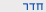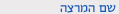שנה"ל תש"ףמכניקה קלאסית להנדסת חשמל
Classical Mechanics for Electrical Engineering1
0509-1118-11
הנדסה | תואר ראשון - קורסי שירותסמ'  ב' 1300-1500 'ה 103 הנדסת תוכנה תרגיל מר קרניאלי אביב
סילבוס מקוצר
וקטורים,קינמטיקה,חוקי ניוטון, שמור תנע קווי,עבודה ואנרגיה קינטית,שימור אנרגיה, מערכות לא אינרציאליות, תנע זוויתי, מומנטים, שימור תנע זוויתי, דינאמיקה של מערכות חלקיקים, תנועה הרמונית, דינאמיקה של גוף קשיח, תנועה בשדה כובד.
Course description
1. Introduction: physical units, accuracy of experiments and approximations in theories, vectors and scalars, addition of vectors, size of a vector, vectors’ components, representations of vectors, scalar and vector products.
2. Kinematics: motion in one dimension, acceleration, speed and velocity. Motion in three dimensions, Circular motion 1, Acceleration and velocity in polar coordinates.
3. Galilean transformations and relative motion, rotating frame of reference.
4. Newton ’s’ Laws, Momentum and conservation of momentum, inertial mass, friction, motion in a fluid.
5. System with variable mass, rocket motion.
6. Work and energy, Definition of work, path integral, power, kinetic and potential energy, conservative forces, conservation of energy, central force, non-conservative forces.
7. Non-inertial frame of reference, rotating frame of reference, motion relative to earth 2.
8. Angular momentum, Conservation of angular momentum, motion under the influence of a central force. statics: equilibrium of a particle, center of mass, equilibrium of a rigid body.
9. Dynamics of a system of particles, motion in the center of mass frame of reference, angular momentum and energy in the center of mass frame of reference, collisions, two particle interactions.
10. Dynamics of a rigid body, angular momentum of a rigid body, rotation around a fixed axis, calculation of the moment of inertia.
11. Equations of motion of a rigid body with a fixed axis in space, Energy of rotation, precession, rolling with and without friction.
12. Simple harmonic motion, energy of a harmonic oscillator, simple pendulum, Physical pendulum.
13. Coupled harmonic oscillators, small amplitude oscillations.
14. Damped harmonic oscillator, with and without a harmonic driving force, resonance, impedance, quality factor.
15. Motion in a gravitational field, Newton ’s laws of gravity, gravitational mass, motion under a gravitational interaction, gravitational field and potential.

להצהרת הנגישות